Function Repository Resource:

# SubsetIndex

Give the index of a subset

Contributed by: Ed Pegg Jr
 ResourceFunction["SubsetIndex"][list] gives the index of subset list.

## Details and Options

Subsets are considered to be lists {a1,a2,,ak} such that the ai are non-negative integers and strictly increasing.
Subsets are sorted by their reverse; {1,2,3} comes before {0,1,4}.
For any subset, a unique index n can be defined via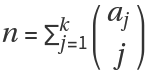.
For example: subset {2,4,6} has index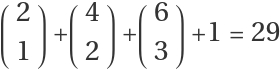.

## Examples

### Basic Examples (3)

Find the index of a tuple:

 In:=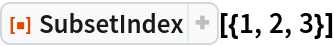Out=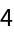The following 3-subset sequence can be extended to infinity:

 In:=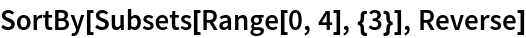Out=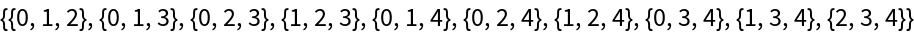The function returns indices of one to ten for these subsets:

 In:=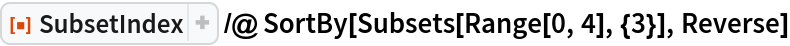Out=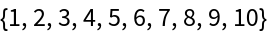Any strictly increasing list of nonnegative integers can be considered as a subset with a unique index:

 In:=Out=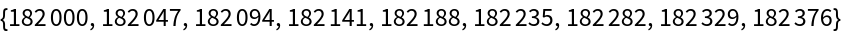The result above is a subset with a unique index:

 In:=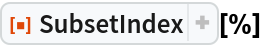Out=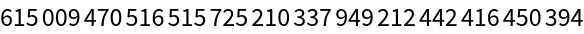### Scope (3)

Various subsets of differing lengths have index 321:

 In:=Out=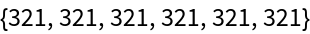Some binomial representations of the number 320:

 In:=Out=Here are some 2-subsets with their indices to show their structure:

 In:=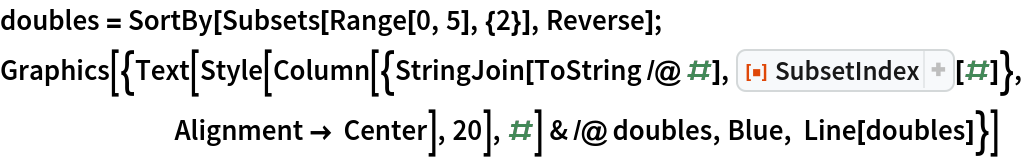Out=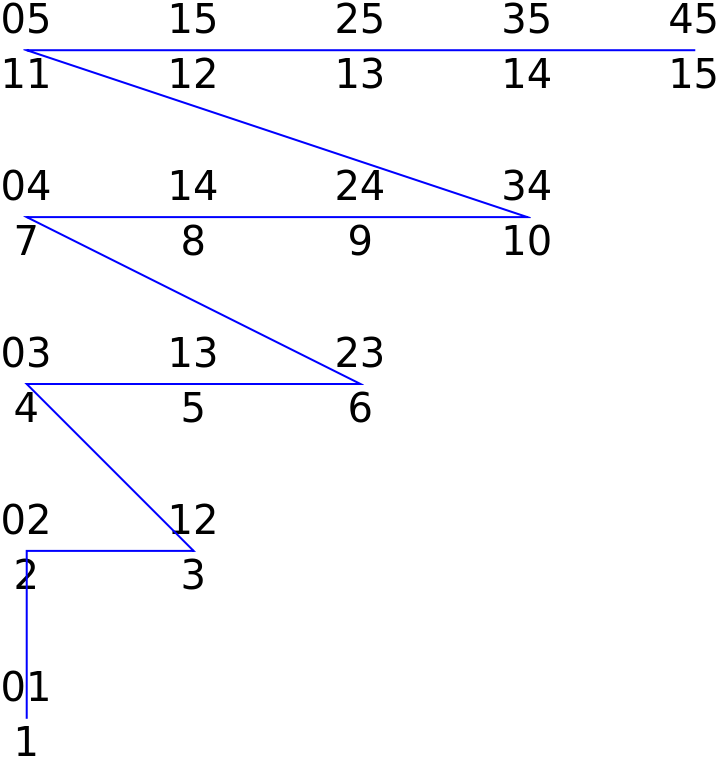The structure of 3-subsets in 3D:

 In:=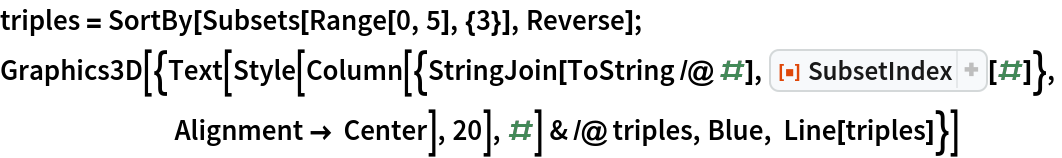Out=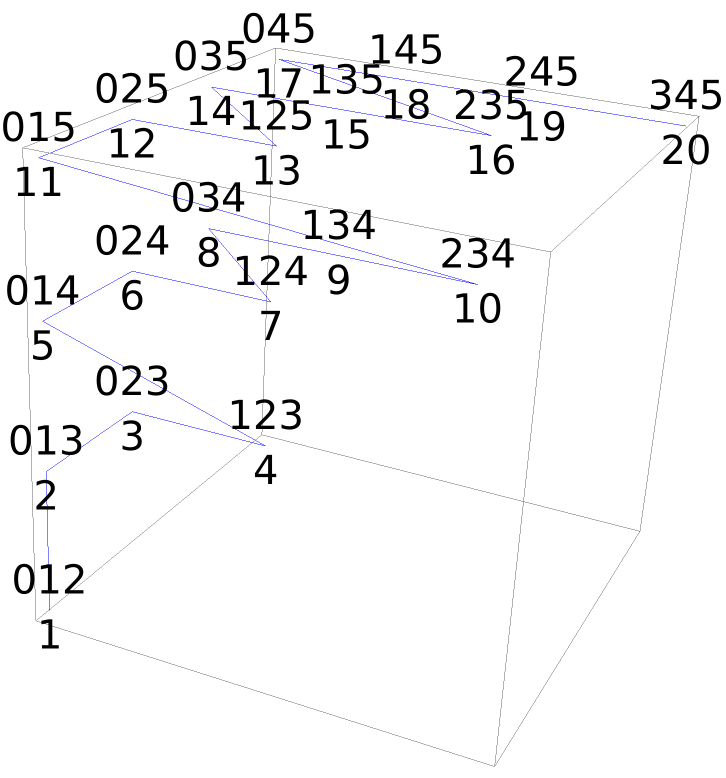### Neat Examples (2)

Find the index of an eight-term subset:

 In:=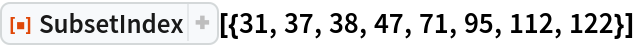Out=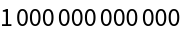From that, we get the trillionth number with binary weight eight:

 In:=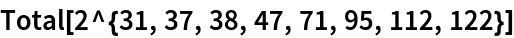Out=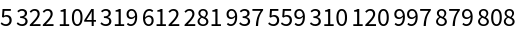## Version History

• 2.0.0 – 26 December 2019
• 1.0.0 – 05 December 2019